# These Approximations are the Foundation for a Hidden Framework in The Mind

In this article I will introduce a series of approximations that are very real, but are only true within a truncated region of space.

Mathematicians have labored for over 6 millenia in order to rid mathematics of 'approximations'.

They want to know as many decimals as possible, in order to be increasingly 'precise' - within our seemingly infinite space.

Approximations are only considered good enough until a new invention comes along that somehow increases the precision of spatial measurement.

Sometimes new tools can turn a measurement from 'approximate' to 'exact'. That's considered an achievement in mathematics.

However, there are many calculations that mathematicians can say for certain will never be 'exact'. These calculations always contain an 'irrational' number.

When calculating with irrational numbers mathematicians use a single strategy - 'More decimals = more precision'. They want to calculate the most precise answers possible using the most precise irrational numbers possible. For example, they have unearthed thousands of digits of PI:

3.14159265358979323846264 3383279502884197169399375 10582097494459230781640628 620899862803482534211706 7982148086513282306647093 84460 9550582231725359408 12848111...

It's very important to consider that it only takes 39 decimals places of PI to calculate the circumference of the entire Universe within the width of a single hydrogen atom! ... This means that calculating thousands and millions of digits of PI is not really useful other than for providing an exercise on 'randomness' and 'infinity'.

So what is the exact value of an irrational number? Nobody can say for sure - therefore mathematicians have a single mindset:

'More decimals = more precision'.

However, their assumptions lay on one particular principle: that they can produce unlimited decimals.

What if only 4 decimals could be produced? What if decimals require 'space', and there are some spaces that just aren't big enough?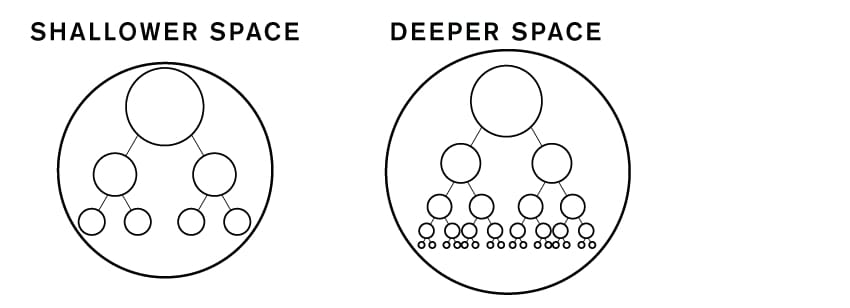If any space can be illustrated within a hierarchical pattern, then each layer represents an additional decimal of precision.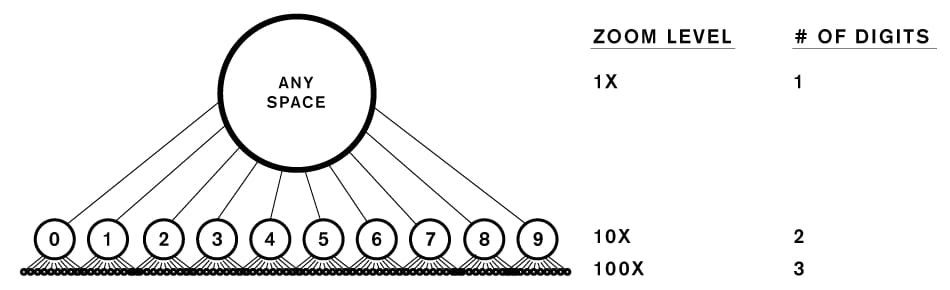Each layer represents 10x more precision in calculating the 'depth' of that space.

This is essential how a ruler works.

But if the space being measured is just 4 layers deep, its numbers could only be measured up to 4 digits (ie. decimals).

That's where these approximations become very precise.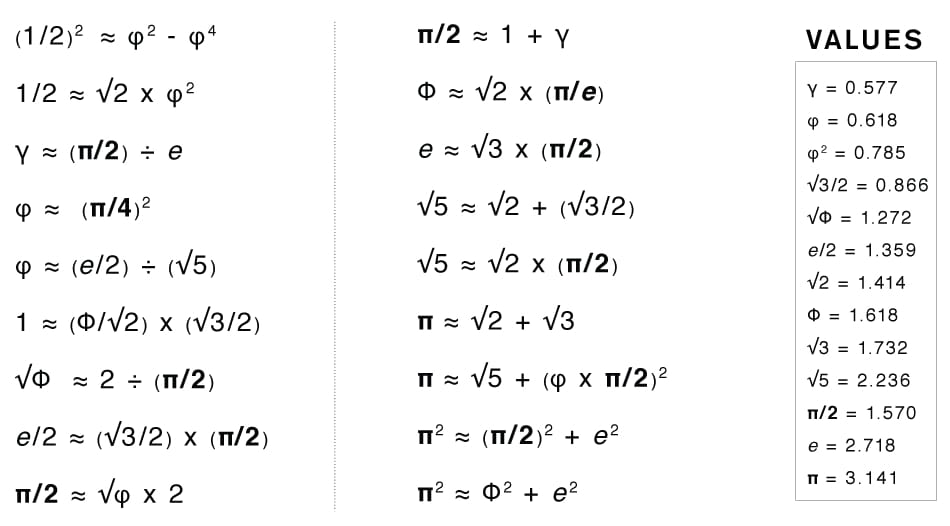All of the equations above are true if the number of digits used in calculations is less than or equal to 4.

The equations include all of the most important irrational constants found in nature.

Notice that there are no 6’s 7’s 8’s or 9’s within any of the equations. It's because these numbers are not really as important as the first six (0, 1, 2, 3, 4, 5).

In fact, only the integers 1,2,3 and 5 are referenced, which are all Fibonacci numbers!

Why truncate each constant to 4 decimals? I believe it's because of the Tetractys...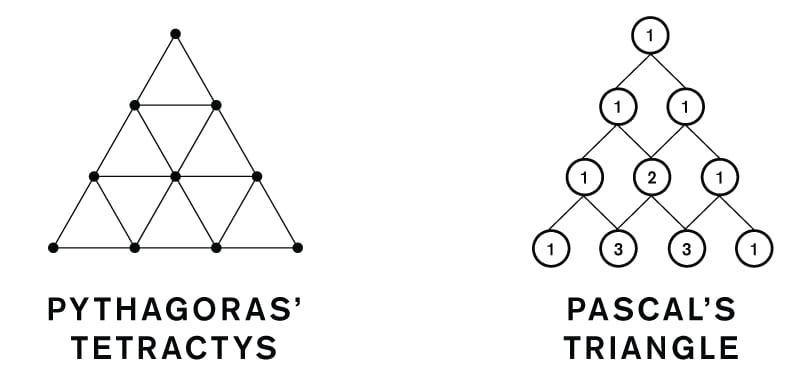The Tetractys represents the first four layers of any mindspace. It's a truncated region, resulting in truncated numbers.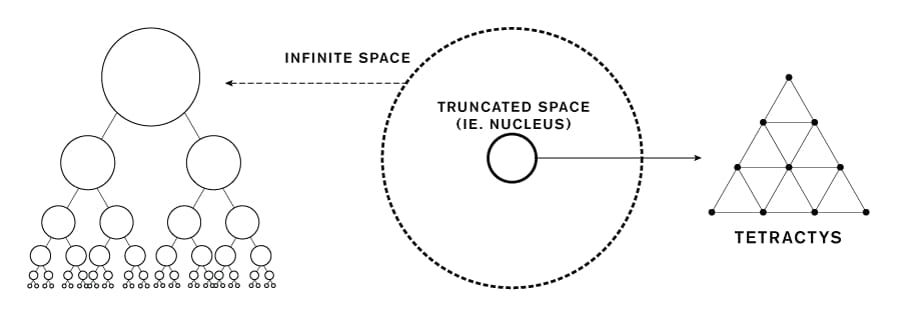It can be compared to a natural capstone sitting on top of every symbol and object in the Universe!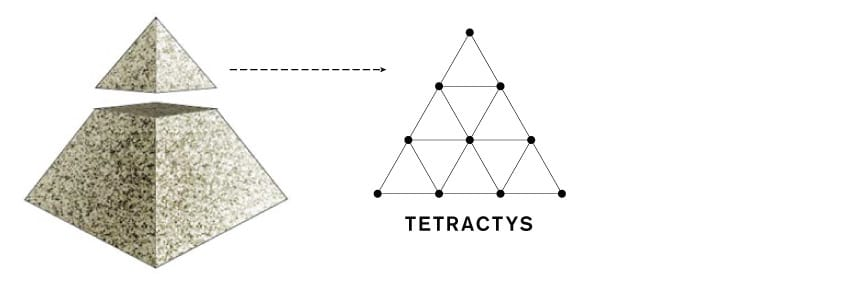Consider that our biological senses function in the range of 100x - 1000x precision. For example, we cannot see a difference between a shape that is drawn in 1,000x or 10,000x (...this is why RETINA displays are about 350ppi, and not 3500ppi).

Phone numbers are usually divided into groups of 3 or 4 numbers, because our minds are naturally tuned to working with 3 to 4 maximum digits.

So this coincidence with our senses may indicate that the spaces we create in our minds are basically founded upon some 4-layer hierarchical patterns.

And these 4-layer patterns are the source for all the most important irrational constants in the Universe because it represents some basic unit of 'depth'.